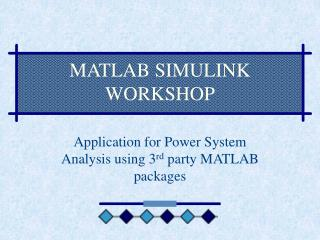DownloadDownload Presentation# MATLAB SIMULINK WORKSHOP

Télécharger la présentation## MATLAB SIMULINK WORKSHOP

- - - - - - - - - - - - - - - - - - - - - - - - - - - E N D - - - - - - - - - - - - - - - - - - - - - - - - - - -
##### Presentation Transcript

1. MATLAB SIMULINK WORKSHOP Application for Power System Analysis using 3rd party MATLAB packages

2. MATPOWER • MATPower is a freeware 3rd party Matlab package written with the objective of solving power flow iterations • Designers of MATPower: • Ray Zimmerman • Deqiang (David) Gan • Both are researchers from the Power System Engineering Research Centre (PSERC) based in the US

3. What is MATPower • It is a collection of matlab m-files to do various types of power flow iterations: • Conventional Static Power Flow – Using Newton Raphson or Gauss Sidel or Fast Decoupled Load flow Iterations • Optimal Power Flow – Using Newton Raphson or Gauss Sidel or Fast Decoupled Optimal Load Flow Iterations

4. Conventional Static Power Flow • As explained earlier, with the objective of calculating the final P Q flow in each branch in the system. • Basic MATPower m-file: • Runpf.m

5. Optimal Power Flow (OPF) • OPF is similar to the traditional PF iterations with the addition of generator scheduling optimisation. • OPF will generate the final P Q flow based upon the optimised generator scheduling with the minimal cost wastage. • Basic MATPower m-file: • Runopf.m

6. Advantages of MATPower • It is a freeware • Its licensing agreement gives the user to access to the source code hence modifying it provided that acknowledgement must be made back to the original author • Runs in Matlab hence reduces the programming constraints due to Matrix sizes and matrix manipulations

7. Advantages of MATPower • In terms of speed for Standard PF, it is fast enough (in the range of a 1-2 seconds, actual speed depending upon the network size). • Results of iteration is in a data form which gives easy manipulation using other programming platforms, e.g. C++, fortran.

8. Disadvantage of MATPower • Iteration speed during optimal power flow decreases as the system size is increased. • This is due to the optimization process which requires optimal generator dispatch. • Results are in the form of data file. • This may be solve by adding a graph plotting m-file or converting the data file into Excel for graphical manipulation.

9. Example of MATPower • In studying the system properties of an IEEE 300 test system as it is loaded into instability. • A C++ program is written to automatically increase the load level in the MATPower input data file. • Format of output iteration is modified to suit with a researched algorithm also written in C++.

10. Excerpt of Simulation Results Bus voltage magnitudes as the system load is increased slowly at all load centres.

11. Excerpts of Simulation Results Bus voltage angle difference with reference to the nominal loading factor at all load centres loading strategy

12. Thank You